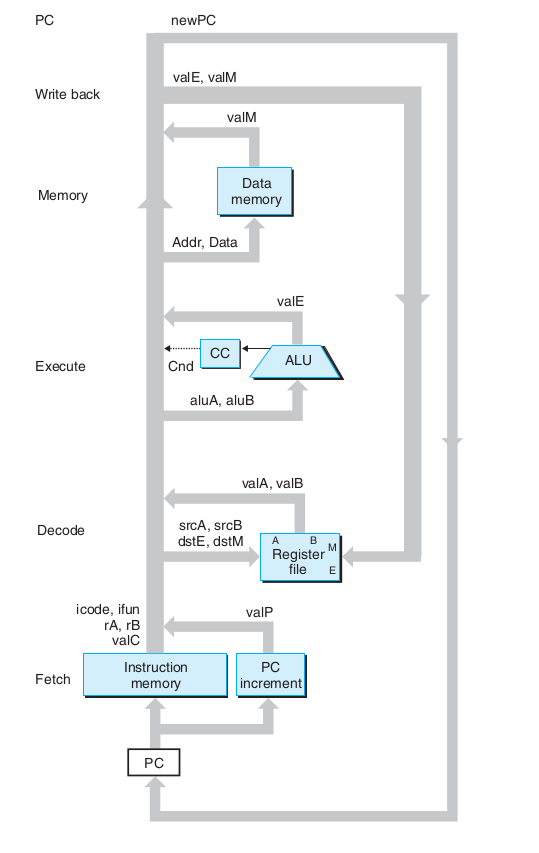# C语言中的int类型的范围是由什么决定的

an integer, typically reflecting the natural size of integers on the host machine

/* Minimum and maximum values a signed int' can hold.  */
#  define INT_MIN   (-INT_MAX - 1)
#  define INT_MAX   2147483647


/* test_add.c */
#include <stdio.h>

int main(void) {
int a = 1;
int b = 2;
int c = a + b;

return 0;
}


   gcc -S test_add.c -o test_add.s


    movl    $1, -12(%ebp) movl$2, -8(%ebp)

movl    -8(%ebp), %eax
movl    -12(%ebp), %edx

movl    %eax, -4(%ebp)
`• int 类型
• 指针（即内存地址）
• 寄存器
• 数据总线
• 地址总线